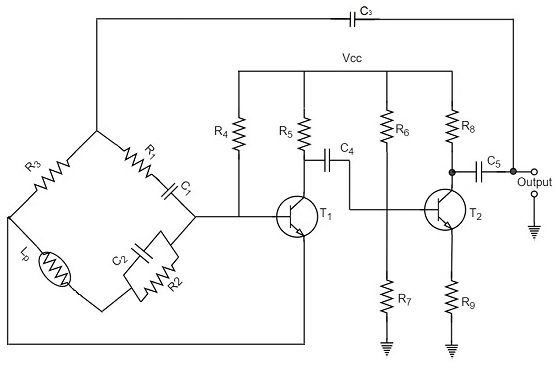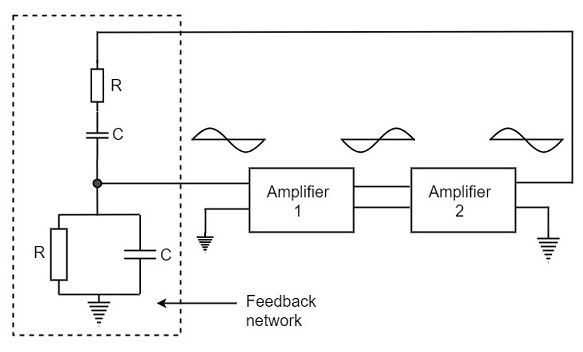# Wien Bridge Oscillator

Another type of popular audio frequency oscillator is the Wien bridge oscillator circuit. This is mostly used because of its important features. This circuit is free from the circuit fluctuations and the ambient temperature.

The main advantage of this oscillator is that the frequency can be varied in the range of 10Hz to about 1MHz whereas in RC oscillators, the frequency is not varied.

## Construction

The circuit construction of Wien bridge oscillator can be explained as below. It is a two-stage amplifier with RC bridge circuit. The bridge circuit has the arms R1C1, R3, R2C2 and the tungsten lamp Lp. Resistance R3 and the lamp Lp are used to stabilize the amplitude of the output.

The following circuit diagram shows the arrangement of a Wien bridge oscillator.The transistor T1 serves as an oscillator and an amplifier while the other transistor T2 serves as an inverter. The inverter operation provides a phase shift of 180o. This circuit provides positive feedback through R1C1, C2R2 to the transistor T1 and negative feedback through the voltage divider to the input of transistor T2.

The frequency of oscillations is determined by the series element R1C1 and parallel element R2C2 of the bridge.

$$f = \frac{1}{2 \pi \sqrt{R_1C_1R_2C_2}}$$

If R1 = R2 and C1 = C2 = C

Then,

$$f = \frac{1}{2\pi RC}$$

Now, we can simplify the above circuit as follows −The oscillator consists of two stages of RC coupled amplifier and a feedback network. The voltage across the parallel combination of R and C is fed to the input of amplifier 1. The net phase shift through the two amplifiers is zero.

The usual idea of connecting the output of amplifier 2 to amplifier 1 to provide signal regeneration for oscillator is not applicable here as the amplifier 1 will amplify signals over a wide range of frequencies and hence direct coupling would result in poor frequency stability. By adding Wien bridge feedback network, the oscillator becomes sensitive to a particular frequency and hence frequency stability is achieved.

## Operation

When the circuit is switched ON, the bridge circuit produces oscillations of the frequency stated above. The two transistors produce a total phase shift of 360o so that proper positive feedback is ensured. The negative feedback in the circuit ensures constant output. This is achieved by temperature sensitive tungsten lamp Lp. Its resistance increases with current.

If the amplitude of the output increases, more current is produced and more negative feedback is achieved. Due to this, the output would return to the original value. Whereas, if the output tends to decrease, reverse action would take place.

The advantages of Wien bridge oscillator are as follows −

• The circuit provides good frequency stability.

• It provides constant output.

• The operation of circuit is quite easy.

• The overall gain is high because of two transistors.

• The frequency of oscillations can be changed easily.

• The amplitude stability of the output voltage can be maintained more accurately, by replacing R2 with a thermistor.